Subtraction of fractionsCustom SearchSUBTRACTION The rule of likeness applies in the subtraction of fractions as well as in addition. Some examples will show that cases likely to arise may be solved by use of ideas previously developed.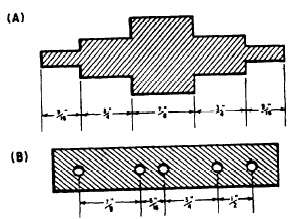Figure 4-5.-Adding fractions to obtain total length or spacing. EXAMPLE: Subtract 1 1/3 from 5 2/3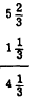We see that whole numbers are subtracted from whole numbers; fractions from fractions. EXAMPLE: Subtract 1/8 from 4/5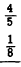Changing to like fractions with an LCD, we have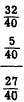EXAMPLE: Subtract 11/12 from 3 2/3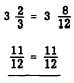Regrouping 3 8/12 we haveThenPractice problems. Subtract the lower number from the upper number and reduce the difference to simplest terms: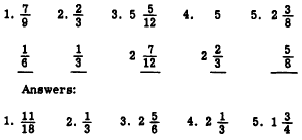The following problem demonstrates subtraction of fractions in a practical situation. EXAMPLE: What is the length of the dimension marked X on the machine bolt shown in figure 4-8 (A)? SOLUTION: Total the lengths of the known parts.Subtract this sum from the overall length.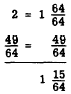The answer is 1 15/64 inchFigure 4-6.-Finding unknown dimensions by subtracting fractions. Practice problem. Find the length of the dimension marked Y on the machine bolt in figure 4-6 (B). Answer 2 3/32 inchesIntegrated Publishing, Inc. - A (SDVOSB) Service Disabled Veteran Owned Small Business